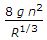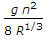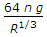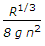# Civil Engineering - UPSC Civil Service Exam Questions

6.

Consider the following limitations :
1. Can be performed only on purely cohesionless soils
2. Plane of failure is predetermined
3. There is virtually no control on drainage
4. Non-uniform distribution of stresses
5. Principal stresses in the sample cannot be determined The limitations inherent in direct shear test include

 A. 1, 2 and 3 B. 2, 3 and 4 C. 3, 4 and 5 D. 1, 2 and 5

Explanation:

No answer description available for this question. Let us discuss.

7.

Given that g = acceleration due to gravity and R = hydraulic mean depth, the Darcy Weisbach friction factor is related to Manning's rugosity coefficient 'n' as

 A.B.C.D.Explanation:

No answer description available for this question. Let us discuss.

8.

A symmetrical circular arch of span 25 m with a central rise of 5 is hinged at crown and springings. It carries a point load on the arch in the span between the left hinge and central hinge. If the inclination of the thrust at the right hinge is θ measured from horizontal, then what is the value of tan θ ?

 A. 2.5 B. 1.0 C. 0.4 D. Not possible to calculate with given data

Explanation:

No answer description available for this question. Let us discuss.

9.

The combined motion of rotation and translation may be assumed to be a motion of pure rotation about some centre which goes on changing from time to time. The centre in question is known as

 A. Shear centre B. Meta centre C. Instantaneous centre D. Gravitational centre

Explanation:

No answer description available for this question. Let us discuss.

10.

A train is hauled by 2-8-2 locomotive with 22.5 tonnes load on each driving axle. Assuming the coefficient of rail-wheel friction to be 0.25, what would be the hauling capacity of the locomotive ?

 A. 15.0 tonnes B. 22.5 tonnes C. 45.0 tonnes D. 90.0 tonnes